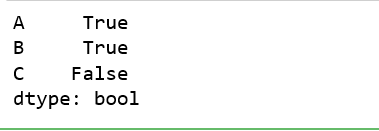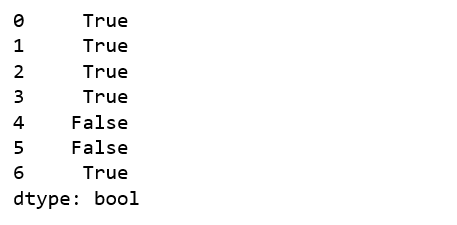Related Articles

# Python | Pandas Series/Dataframe.any()

• Last Updated : 16 Oct, 2018

Python is a great language for doing data analysis, primarily because of the fantastic ecosystem of data-centric python packages. Pandas is one of those packages and makes importing and analyzing data much easier.

Pandas `any()` method is applicable both on Series and Dataframe. It checks whether any value in the caller object (Dataframe or series) is not 0 and returns True for that. If all values are 0, it will return False.

Syntax: DataFrame.any(axis=0, bool_only=None, skipna=True, level=None, **kwargs)

Parameters:
axis: 0 or ‘index’ to apply method by rows and 1 or ‘columns’ to apply by columns.
bool_only: Checks for bool only series in Data frame, if none found, it will use only boolean values. This parameter is not for series since there is only one column.
skipna: Boolean value, If False, returns True for whole NaN column/row
level: int or str, specifies level in case of multilevel

Return type: Boolean series

Example #1: Index wise implementation

In this example, a sample data frame is created by passing dictionary to Pandas `DataFrame()` method. Null values are also passed to some indexes using Numpy `np.nan` to check behaviour with null values. Since in this example, the method is implemented on index, the axis parameter is kept 0 (that stands for rows).

 `# importing pandas module ``import` `pandas as pd `` ` `# importing numpy module``import` `numpy as np`` ` `# creating dictionary``dic ``=` `{``'A'``: [``1``, ``2``, ``3``, ``4``, ``0``, np.nan, ``3``],``       ``'B'``: [``3``, ``1``, ``4``, ``5``, ``0``, np.nan, ``5``],``       ``'C'``: [``0``, ``0``, ``0``, ``0``, ``0``, ``0``, ``0``]}`` ` `# making dataframe using dictionary``data ``=` `pd.DataFrame(dic)`` ` `# calling data.any column wise``result ``=` `data.``any``(axis ``=` `0``)`` ` `# displaying result``result`

Output:
As shown in output, since last column is having all values equal to zero, Hence False was returned only for that column.Example #2: Column wise Implementation

In this example, a sample data frame is created by passing dictionary to Pandas `DataFrame()` method just like in above example. But instead of passing 0 to axis parameter, 1 is passed to implement for each value in every column.

 `# importing pandas module ``import` `pandas as pd `` ` `# importing numpy module``import` `numpy as np`` ` `# creating dictionary``dic ``=` `{``'A'``: [``1``, ``2``, ``3``, ``4``, ``0``, np.nan, ``3``],``       ``'B'``: [``3``, ``1``, ``4``, ``5``, ``0``, np.nan, ``5``],``       ``'C'``: [``0``, ``0``, ``0``, ``0``, ``0``, ``0``, ``0``]}`` ` `# making dataframe using dictionary``data ``=` `pd.DataFrame(dic)`` ` `# calling data.any column wise``result ``=` `data.``any``(axis ``=` `1``)`` ` `# displaying result``result`

Output:
As shown in the output, False was returned for only rows where all values were 0 or NaN and 0.Attention geek! Strengthen your foundations with the Python Programming Foundation Course and learn the basics.

To begin with, your interview preparations Enhance your Data Structures concepts with the Python DS Course. And to begin with your Machine Learning Journey, join the Machine Learning – Basic Level Course

My Personal Notes arrow_drop_up